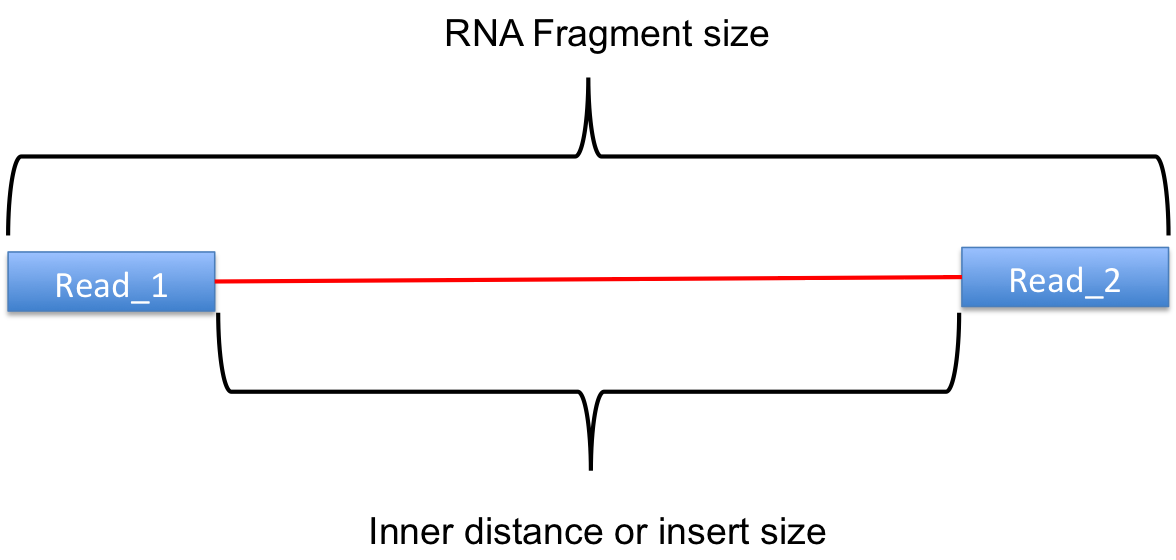Question: the defination of insert size in RNA-seq, including the length of read, or not?
1
zju.whw40 wrote:

In paired-end RNA-seq analysis, some tools require the insert size (also called inner distance) as the parameter to run (such as MATS's -r option). And I also know that some tools can be used to estimate the insert size, such as CollectInsertSizeMetrics in the picard or inner_distance.py in the RSeQC.

I used both the two tools for my 2*100bp RNA-seq data, however, the results are different, as shown Figure1 in the bellow. It seems the distributions are same, but the values of average insert size are different. It seems that it is because, the CollectInsertSizeMetrics calculates the length of template (the RNA fragment), in contrast, the inner_distance.py calculates the length of template minuse the length of two reads (as shown in Figure2 below, source from RSeQC website).

Is there anyone know the defination of insert size in RNA-seq? It should inculde the read length (the method of CollectInsertSizeMetrics), or not (the method of inner_distance.py)?

the mean value of CollectInsertSizeMetrics is 175.825835bp

the mean value of inner_distance.py is −38.1662853494061

(I don't know how to upload a figure into the biostars here.)

Figure1 the mean value and distribution of insert size for my paired-end RNAseq analysisFigure2 the insert size that inner_distance.py calculates, source for RSeQC website.

modified 5.3 years ago • written 5.3 years ago by zju.whw40
3
thackl2.8k wrote:

```---------->         <----------
|_______   insert size ________|```

"Remember that "insert" refers to the DNA fragment between the adaptors, and not the gap between R1 and R2." (http://thegenomefactory.blogspot.de/2013/08/paired-end-read-confusion-library.html)

So the inner_distance.py in the RSeQC is wrong?

1

It's terminology. Inner distance distance refers to the gap between reads. The label in your figure is bad.

```---------->         <----------
|_______   insert size ________|
|__________|
inner distance```